# Chapter 12 - Organic Chemistry: Some Basic Principles and Technique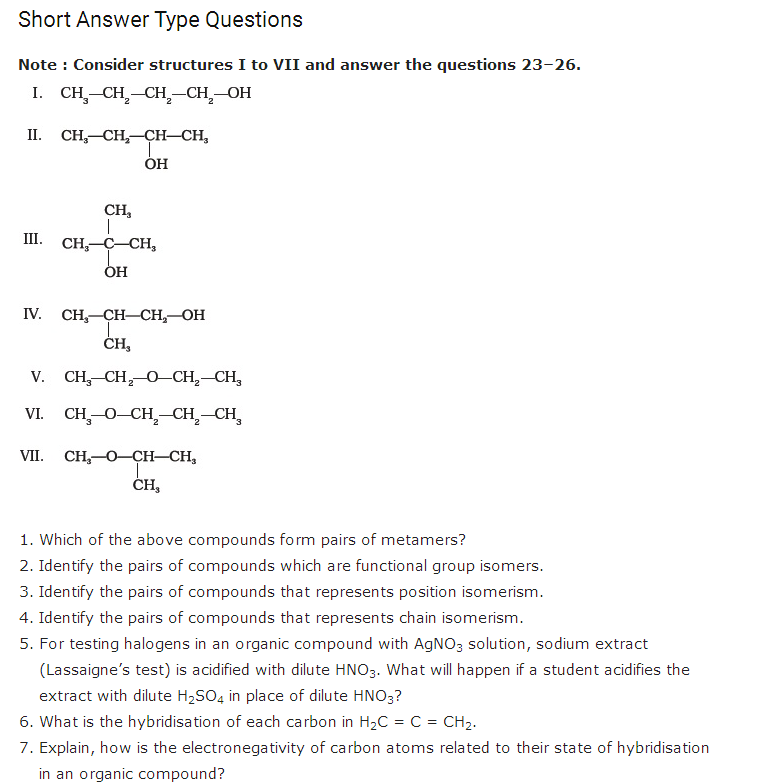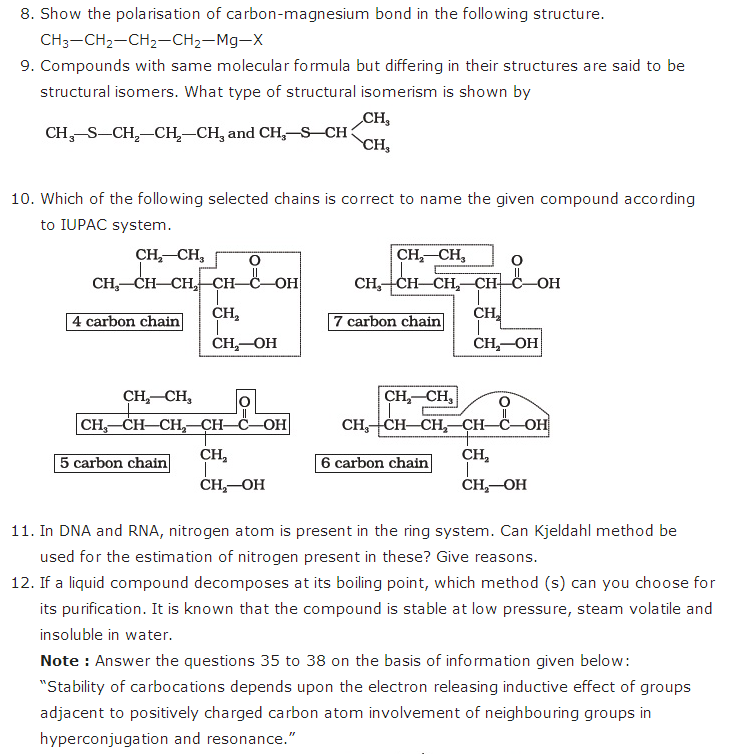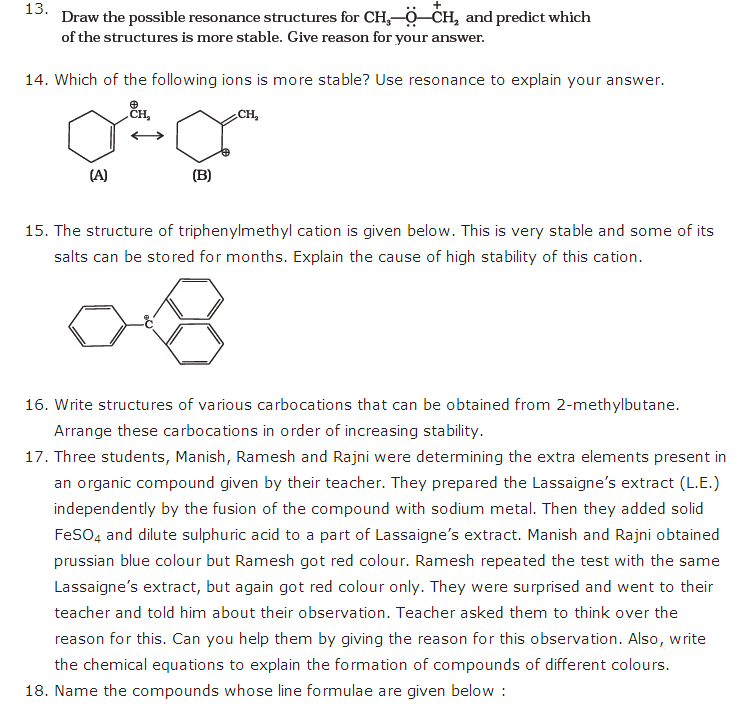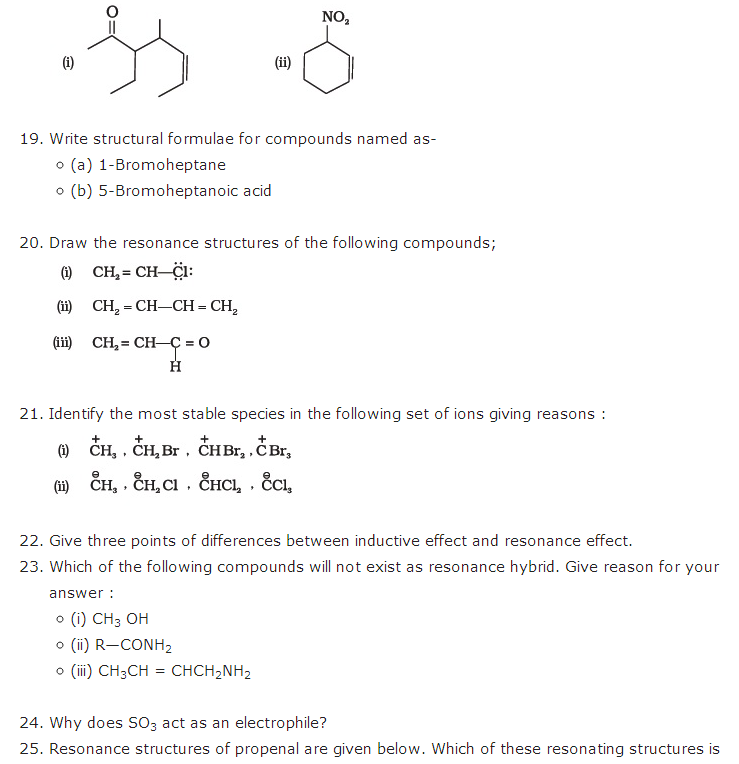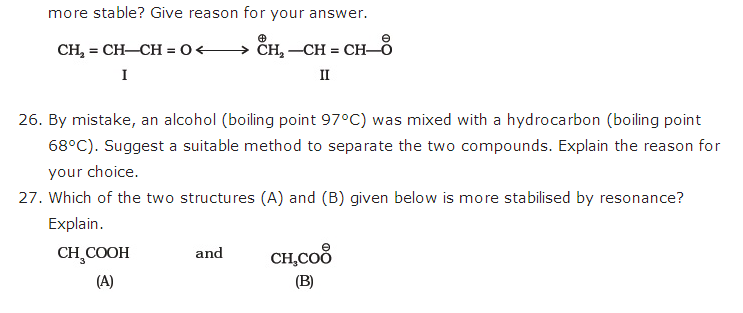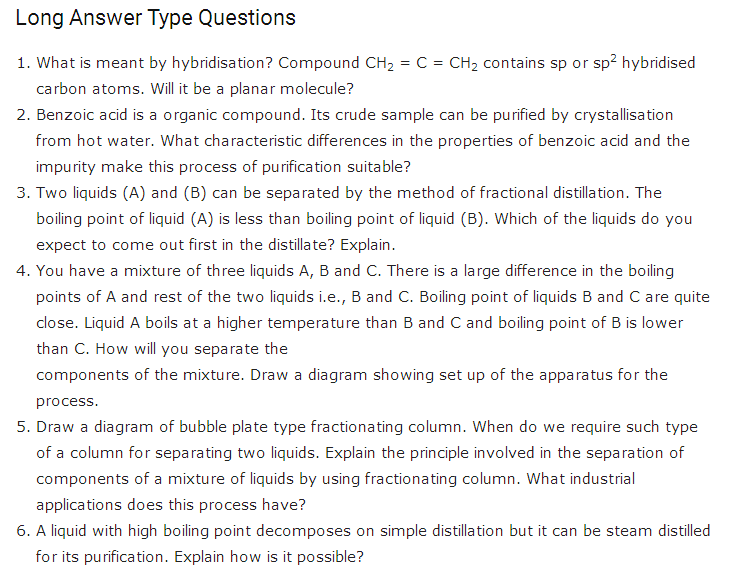#### Practise This Question

The wave function, Ψn,l,ml is a methematical function whose value depends upon spherical polar coordinates (r, θ, Ф) of the electron and characterized by the quantum numbers n, l and m. Here r is distance from nucleus, θ is colatitude and Ф is azimuth. In the mathematical functions given in the Table, Z is atomic number and a0 is Bohr radius.

 Column 1 Column 2 Column 3 (i) 1s orbital (i) Ψn,l,ml∝(Za0)32e(−Zra0) (P)(ii) 2s orbital (ii) One radial node (Q) Probability density at nucleus ∝1a30 (iii) 2 pz orbital (iii) Ψn,l,ml∝(Za0)52re(−Zr2a0)cosθ (R) Probability density is maximum at nucleus (iv) 3d2z orbital (iv) xy-plane is a nodal plane (S) Energy needed to excite electron from n = 2 state to n = 4 state is2732 times the energy needed to excite electron from n = 2 state to n = 6 state

For hydrogen atom, the only CORRECT combination is